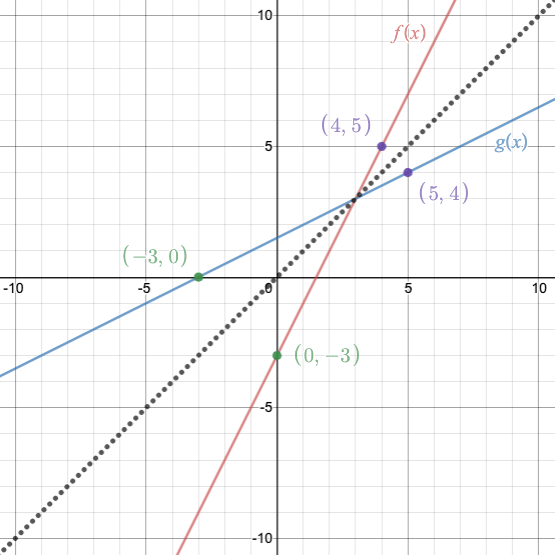# Function Reflections

The graph below contains the graphs of two linear functions that are reflections over the line  $$y = x$$  with  $$f(x) = 2x~–~3$$  and  $$g(x) = \dfrac x2 + \dfrac 32$$ . Graph is created using Desmos.com.1. Identify the location of the point of intersection between the two functions and describe why the location makes sense.
2. Describe the algebraic relationship between the sets of points identified on each figure.
3. Name one more set of points with the same relationship described in Part A.
4. Connect each set of points on each figure. What is the relationship between the line drawn from each point and the line  $$y=x$$ ? What do you notice about the location of each set of points in relationship to the line  $$y=x$$ ?